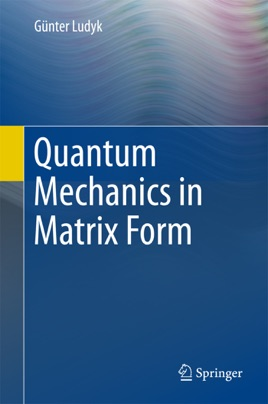• USD 109.99

## Descripción de editorial

This book gives an introduction to quantum mechanics with the matrix method. Heisenberg's matrix mechanics is described in detail. The fundamental equations are derived by algebraic methods using matrix calculus. Only a brief description of Schrödinger's wave mechanics is given (in most books exclusively treated), to show their equivalence to Heisenberg's matrix  method. In the first part the historical development of Quantum theory by Planck, Bohr and Sommerfeld is sketched, followed by the ideas and methods of Heisenberg, Born and Jordan. Then Pauli's spin and exclusion principles are treated. Pauli's exclusion principle leads to the structure of atoms. Finally, Dirac´s relativistic quantum mechanics is shortly presented. Matrices and matrix equations are today easy to handle when implementing numerical algorithms using standard software as MAPLE and Mathematica.